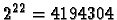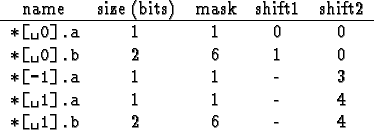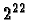Next: 4.3 Coding as a Up: 4. Efficient simulation of Previous: 4.1 Translating CDL to   Contents

Subsections

# 4.2 Table-lookup coding

A cellular automaton in the classical definition is a regular lattice of cells, each of which contains a state selected from a finite set of states. The cells are updated according to a state transition function depending on a finite number of neighbors of each cell. Since both the set of states and the neighborhood are finite, the number of different possible local configurations which can occur as inputs to the state transition function is also finite. If this number is small enough, we can store the result of the local state transition function in a table, and use this table in the simulation instead of the (translated) transition function.

The translation process proceeds along the following steps in the CASim system:

## 4.2.1 Determine active neighborhood

First, the system collects the list of variables used as inputs to the state transition function. These are just those components of the state of the cell and of its neighbors, which appear as read-accesses in the transition function. In many cases the relevant configuration does not contain all parts of the state for all neighbors, but just one or a few components of a neighbor's state.

## 4.2.2 Calculate size of input configuration

From the list of accessed (neighboring) state variables the size of the input configuration is determined: For each distinct part, the number of bits used to store this state component are calculated, and these numbers are added for all parts of the active neighborhood. If the result is at most 22 bits, this means that the look-up table has at mostentries, which makes it feasible to store the entire table, and also makes it possible to store the entire state of a cell in one integer variable.

## 4.2.3 Generate masks and shifts for neighbor components

In order to use a look-up table, a method must be found to calculate an index into the table (address) from the local configuration. For the calculation, each active neighborhood component is assigned a bit-mask and a shift for composition into an address. For the example T1 from section 4.1, the following properties are calculated:Here shift1 is the bit position of this component in the integer representation of the state. shift2 is the shift needed to place the bit pattern masked by the mask into the address for access to the look-up table.

The address is calculated as the disjunction of expressions of the form (name & mask )<< shift2 for each component of the active neighborhood, in this example:


int adr = (* & 7)
| ((*[-1] & 1)<<3)
| ((*[ 1] & 1)<<4)
| ((*[ 1] & 6)<<4);

where the first line collects all relevant components of the cell itself, and the other lines refer to the neighbors.

## 4.2.4 Fill the table

To fill the look-up table, we use the state transition function translated into Java in the normal way. We set up a special Cell object which is given an index of the table and provides the appropriate states as neighbors. The transition-method of the original java class is then called once to update the state. The resulting state is converted into an integer (using the mask and local shifts calculated before), and stored in the table. Even though this process must be performed for each table entry, it takes at most a few seconds.

## 4.2.5 Generate Java code to use the table

Finally, a new Java class is generated which uses the look-up table in the state transition function, but otherwise behaves exactly as before (e.g., for initialization and display). This is achieved by subclassing the previously translated state-class. The transition-method is overridden, and additional methods are provided for conversion between the representation using one integer and the representation using seperate variables for the state components.

Example:


public class T4Table extends T4 {
protected int mys;

static final int table[] = {
0,0,2,2,4,4,6,6,0,1,2,3,4,5,6,7
.......
,1,1,3,3,5,5,7,7,1,6,3,6,5,0,7,0
};

public void transition(Cell cell){
final State [] neighbors = cell.getNeighbors();
int adr = (mys & 7)
| ((((T4Table)neighbors).mys & 1)<<3)
| ((((T4Table)neighbors).mys & 1)<<4)
| ((((T4Table)neighbors).mys & 6)<<4)
;
mystatetable = table[adr];
}
private void toOriginal(){
mystate.a = (((mys & 1) >> 0)==1);
mystate.b = (((mys & 6) >> 1));
}
private void toTable(){
mys = ((mystate.a?1:0) << 0)
| ((mystate.b) << 1) ;
}
.....


## 4.2.6 Limitations

The translation from CDL and Java code into the table form has a number of limitations. Most importantly, the size of the active neighborhood may not be too large, since tables targer than aboutentries are impractical to generate and to store. A second limitation is that probabilistic rules are difficult to handle. If only one binary probabilistic choice appears in the transition function, and this choice has a fixed probability (as opposed to a data-dependent probability), then thi schoice can be incorporated as an extra input bit in th etransition table, and a bit with the appropriate statistics can be generated for each table-lookup operation. This approach only extends to a few binary choices or one probabilistic choice with very few alternatives and no data dependency. Otherwise, the table would become prohibitively large.

Obviously, the table method is not applicable to cell states that contain floating-point numbers or integers without range limitation.Next: 4.3 Coding as a Up: 4. Efficient simulation of Previous: 4.1 Translating CDL to   Contents
Jörg R. Weimar